• 分析了Kobara和Imai基于信息论观点给出的该类窄带阈下信道实际可达信道容量的定义,指出这种容量的定义方式没有考虑单次通信时的误码率问题,而这对阈下通信环境非常重要。针对基于信息论的容量定义的不足,给出了基于...
• 讨论思路信道容量定义及物理意义定义物理意义（David 无线通信基础）AWGN信道下信道容量AWGN信道（离散时间）信道容量：平坦衰落信道的容量CDI 已知CSIR各态历经容量内容与AWGN容量的关系使用场景中断容量内容适用...
无线信道的信道容量总论来源：讨论思路信道容量定义及物理意义定义物理意义（David 无线通信基础）AWGN信道下信道容量AWGN信道（离散时间）信道容量：平坦衰落信道的容量CDI 已知CSIR各态历经容量内容与AWGN容量的关系使用场景中断容量内容适用场景CSIRT系统模型功率控制最优功率控制（注水法）求解物理意义次优功率控制（信道反转）各容量对比频率选择性信道时不变时变
总论
来源：

Andrea Goldsmith-Wireless Communication
David Tse-Fundamentals of Wireless Communication

讨论思路
首先从定义和物理意义角度讨论无线信道的容量是什么，此处内容主要来自于 David Tse-Fundamentals of Wireless Communication。
之后讨论在不同信道以及不同通信场景下，信道容量的值，主要有两个维度：

不同信道（AWGN信道、平稳时变信道、频率选择性时不变以及时变信道）
不同通信场景（收端知信道分布信息CDI、收端知信道信息CSIR、收发都知CSIRT）

信道容量定义及物理意义
定义
1、当误码率接近无限小时，通信系统所允许的最高传输速率。
$C=max(R_{\mathrm{b}}|BER \rightarrow 0)$
2、信道输入与输出的互信息量的最大值。
$C=\text{max }I(\text{input;output})$
物理意义（David 无线通信基础）
根据采样定理，任何一个带宽为$B$，时长为$T$的信号，至少需要$N=2BT$个样本才能重建，所以信号空间为$N$维，所以任意一个信号可以看成是$N$维欧氏空间中的一个点。
假设给定发送信号功率约束$P$,高斯白噪声的方差为$\sigma^{2}$，根据大数定律，$N$维接受矢量将高概位于半径为$\sqrt{N{P+\sigma^2}}$的超球内，而考虑噪声的影响，对于发射星座点，对应接受矢量应高概位于半径为$\sqrt{N}\sigma$的噪声球附近。如果要无误通信，相邻噪声球不应该重叠，所以物理意义是将多个噪声球填充进入$N$维信号空间的超球中，最大填充个数则是我们的信道容量：
$\frac{\sqrt{N\left(P+\sigma^{2}\right)}^{N}}{\sqrt{N \sigma^{2}} N}$
比特化后有：
$\frac{1}{T} \log \left(\frac{\sqrt{N\left(P+\sigma^{2}\right)}^{N}}{\sqrt{N \sigma^{2}} N}\right)=B \log \left(1+\frac{P}{\sigma^{2}}\right)$
物理意义图示如下：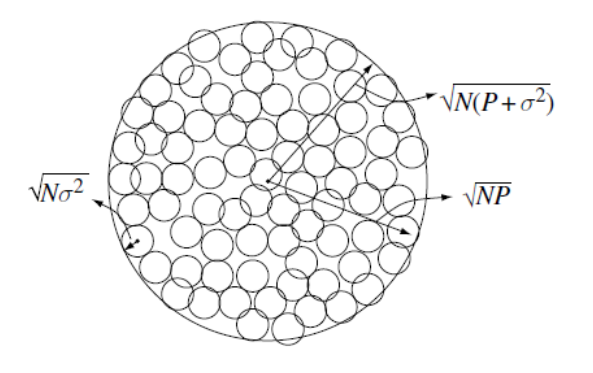此处推荐知乎回答
AWGN信道下信道容量
AWGN信道（离散时间）
$y[i]=x[i]+n[i]$
AWGN信道无衰落，所以接受信噪比是恒定值$\gamma=P /\left(N_{0} B\right)$
信道容量：
$C=B \log _{2}(1+\gamma)$
容量最大输入是高斯分布。
香农容量是数据率的上界。
平坦衰落信道的容量
在AWGN基础上加入了平坦衰落，时变的信道增益系数为$\sqrt{g[i]}, 0 \leq g[i]$，系统模型为：
$y[i]=\sqrt{g[i]} x[i]+n[i]$
系统框图：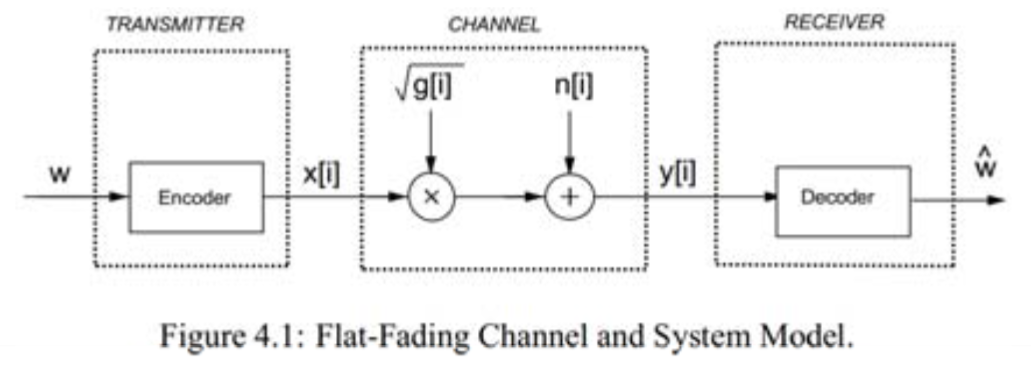信道功率增益系数$g[i]$服从分布$p(g)$，则接受信噪比为：
$\gamma[i]=\bar{P} g[i] /\left(N_{0} B\right), 0 \leq \gamma[i]<\infty$
其中$\bar{P}$为平均发送功率。
对于信道增益的先验信息有三种：

信道分布信息(CDI)已知——收发都已知 $g[i]$的分布；
接收端已知(CSIR)——接收端已知$g[i]$在时刻$i$的值，且收发都已知$g[i]$的分布；
发送端和接收端都已知(CSITR)。

CDI 已知
容量求解，用下式：
$C=\max _{p(x)} I(X ; Y)=\max _{p(x)} \sum_{x, y} p(x, y) \log \left(\frac{p(x, y)}{p(x) p(y)}\right)$
求解困难
CSIR
此时有两种讨论容量的角度：

各态历经容量：平均误码率为零的最大传输速率。
中断容量：在某个中断概率下的信道最大传输速率。

各态历经容量
内容
$C=\int_{0}^{\infty} B \log _{2}(1+\gamma) p(\gamma) d \gamma$
这是一个理论恒定值，即不可将其理解为，当接受信噪比为$\gamma$时，速率为$B \log _{2}(1+\gamma)$，因为发端没有CSI，只能以恒定速率传输
与AWGN容量的关系
\begin{aligned} &\begin{aligned} E\left[B \log _{2}(1+\gamma)\right] &=\int B \log _{2}(1+\gamma) p(\gamma) d \gamma \\ & \leq B \log _{2}(1+E[\gamma])\\ &=B \log _{2}(1+\bar{\gamma}) \end{aligned}\\ \end{aligned}
可见对于相同的平均接受信噪比$\bar{\gamma}$，CSIR的信道容量小于AWGN的信道容量，这说明信道衰落总是会降低容量。
使用场景
快变信道，因为其要求信道编码应该遍历各个信道状态，而慢变信道造成的编码长度太长。
中断容量
在允许一定误码时（出错重传）的信道容量，则与各态历经相比则可以获得更高的信道容量。
内容
由可接受中断概率$p_{out}$给一个最小的接受信噪比$p_{\text {out }}=p\left(\gamma<\gamma_{\min }\right)$，则中断容量为：
$C=B \log _{2}\left(1+\gamma_{\min }\right)$
平均正确接收的信息率为：
$C_{o}=\left(1-p_{\text {out }}\right) B \log _{2}\left(1+\gamma_{\min }\right)$
适用场景
慢变信道
CSIRT
系统模型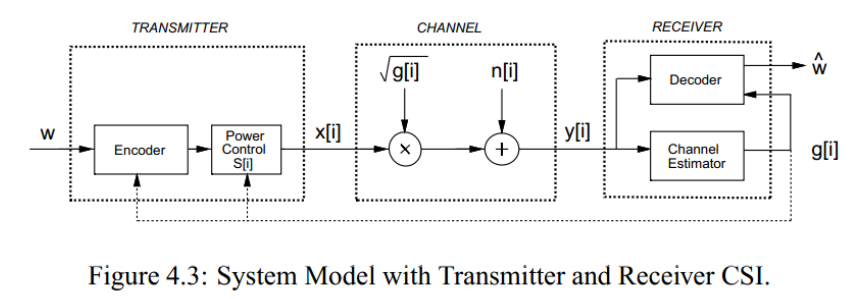CSIT引入最大的不同在于，发端可以控制信息传输速率以及功率控制，进而提供容量增益。
此时信道容量为：
$C=\int_{0}^{\infty} C_{\gamma} p(\gamma) d \gamma=\int_{0}^{\infty} B \log _{2}(1+\gamma) p(\gamma) d \gamma$
从上式可以看出，当CSIRT时，如果不进行功率控制，仅根据接收信噪比调整发送速率，不会增加信道容量，但可以有效提高系统的平均传输速率（使其接近CSIR信道容量）
功率控制
进行功率分配的过程数学化为：
$C=\max _{P(\gamma): \int P(\gamma) p(\gamma) d \gamma=\bar{P}} \int_{0}^{\infty} B \log _{2}\left(1+\frac{P(\gamma) \gamma}{\bar{P}}\right) p(\gamma) d \gamma$
最优功率控制（注水法）
求解
对上式，构造Lagrange：
$J(P(\gamma))=\int_{0}^{\infty} B \log _{2}\left(1+\frac{\gamma P(\gamma)}{\bar{P}}\right) p(\gamma) d \gamma-\lambda \int_{0}^{\infty} P(\gamma) p(\gamma) d \gamma$
令导数为零求极值：
$\frac{\partial J(P(\gamma))}{\partial P(\gamma)}=\left[\left(\frac{B / \ln (2)}{1+\gamma P(\gamma) / \bar{P}}\right) \frac{\gamma}{\bar{P}}-\lambda\right] p(\gamma)=0$
解得最优功控：
$\frac{P(\gamma)}{\bar{P}}=\left\{\begin{array}{ll} \frac{1}{\gamma_{0}}-\frac{1}{\gamma} & \gamma \geq \gamma_{0} \\ 0 & \gamma<\gamma_{0} \end{array}\right.$
其中$\gamma_{0}$为中断门限，表明我们仅在$\gamma_{0} \leq \gamma[i]<\infty$使用信道。且满足：
$\int_{\gamma_{0}}^{\infty}\left(\frac{1}{\gamma_{0}}-\frac{1}{\gamma}\right) p(\gamma) d \gamma=1$
$\gamma_{0}$无闭式解，通过数值解法求解。
将上式代入原信道容量公式，有：
$C=\int_{\gamma_{0}}^{\infty} B \log _{2}\left(\frac{\gamma}{\gamma_{0}}\right) p(\gamma) d \gamma$
物理意义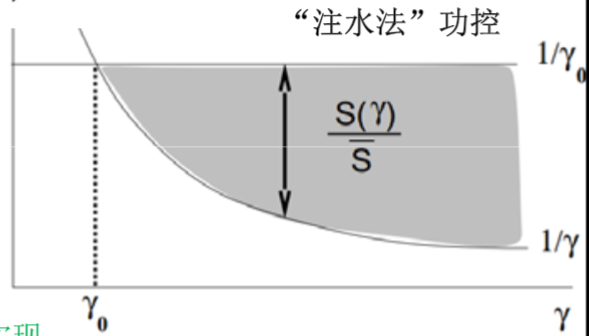$1/\gamma_{0}$为水深，$1/\gamma$为碗深，我们向碗中注水直至水的总体积为总功率。
表明，在数据传输过程中，我们在信道条件好的时候（$\gamma$较大时），多分配功率，且用高速率传输；在信道条件差的时候（$\gamma$较小时），少分配功率，且用低速率传输；如果信道条件太差（低于$\gamma_{0}$），则不进行数据传输。
次优功率控制（信道反转）
恒速率传输，用功控直接抵消信道衰减的影响，使得整个信道等效于AWGN信道。
信道反转由$P(\gamma) / \bar{P}=\sigma / \gamma$确定，$\sigma$是满足功率约束条件下的恒定接收信噪比。
零中断信道容量为：
$C=B \log _{2}[1+\sigma]=B \log _{2}\left[1+\frac{1}{\mathbf{E}[1 / \gamma]}\right]$
上述也是零中断信道反转容量，当考虑中断概率时，只有条件好于中断门限才补偿，否则不传：
$\frac{P(\gamma)}{\bar{P}}=\left\{\begin{array}{ll} \frac{\sigma}{\gamma} & \gamma \geq \gamma_{0} \\ 0 & \gamma<\gamma_{0} \end{array}\right.$
则中断概率为$p_{out}$时吞吐量为：
$C\left(p_{\text {out }}\right)=B \log _{2}\left(1+\frac{1}{\mathbf{E}_{\gamma_{0}}[1 / \gamma]}\right) p\left(\gamma \geq \gamma_{0}\right)$
取中断概率使得上式最大即为最大中断容量：
$C=\max _{\gamma_{0}} B \log _{2}\left(1+\frac{1}{\mathbf{E}_{\gamma_{0}}[1 / \gamma]}\right) p\left(\gamma \geq \gamma_{0}\right)$
其中$\mathbf{E}_{\gamma_{0}}[1 / \gamma] \triangleq \int_{\gamma_{0}}^{\infty} \frac{1}{\gamma} p(\gamma) d \gamma$
各容量对比
下面有对数正态分布，瑞利衰落、Nakagami衰落下容量对比，三种信道衰落剧烈程度递减：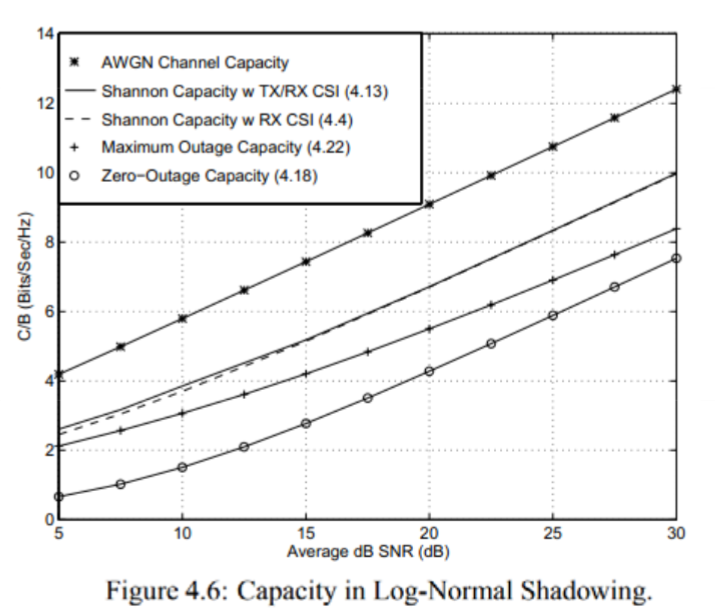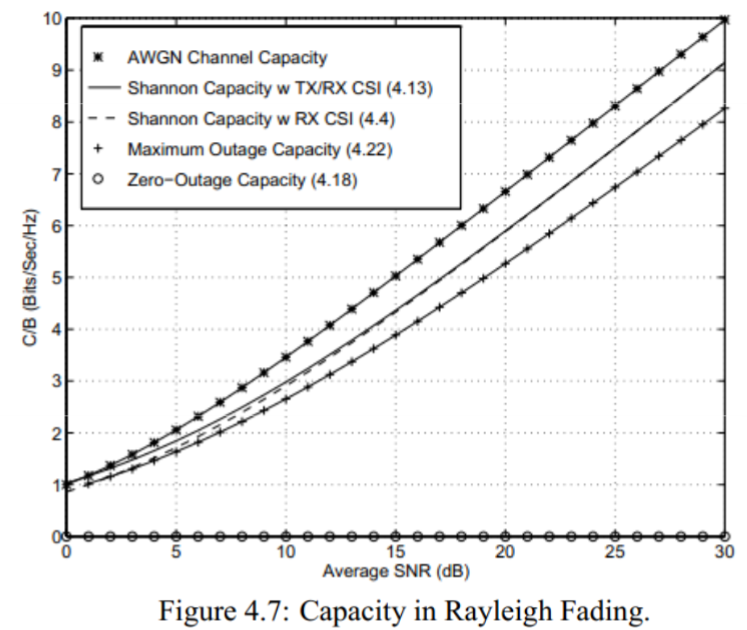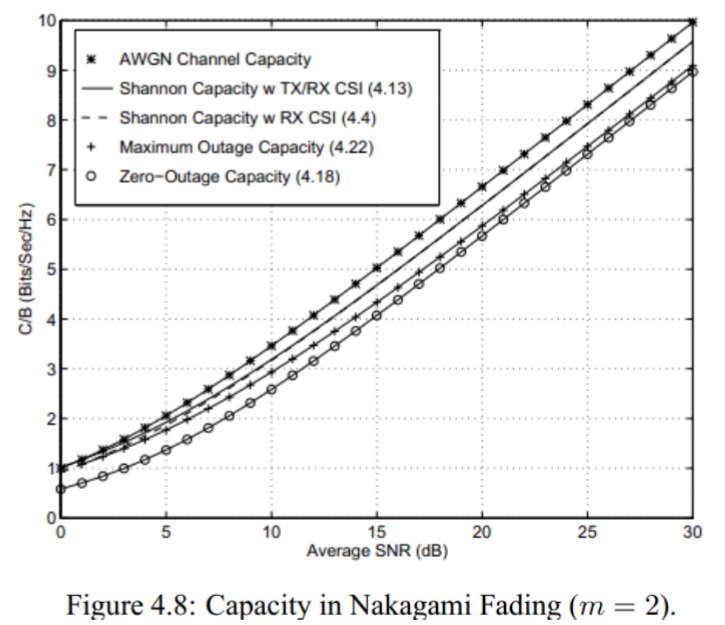如图关注三个问题：

随着信道衰减变得不剧烈（图1->图3），可以看到各个容量逐渐靠近与AWGN信道，且AWGN为任何情况最大容量。
瑞利衰落及Nakagami衰落可以看出，低信噪比下CSIRT接近AWGN甚至大于AWGN，这是由于AWGN是高信噪比下的恒速率传输，当平均信噪比低时，其容量较低，而CSIRT由于是注水法功控，放弃了低信噪比信道而集中功率及速率与偶尔出现的高信噪比信道实现，则会出现容量高于AWGN的情况。
CSIRT相比CSIR，比较靠近且增益不大，这说明信道容量增益主要贡献者是发端的速率控制，功率控制的贡献度不大。

频率选择性信道
基本等同于平台衰落，仅是问题构建不同，这里只讨论CSIRT
时不变
系统框图：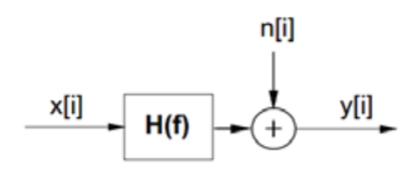按频率切开：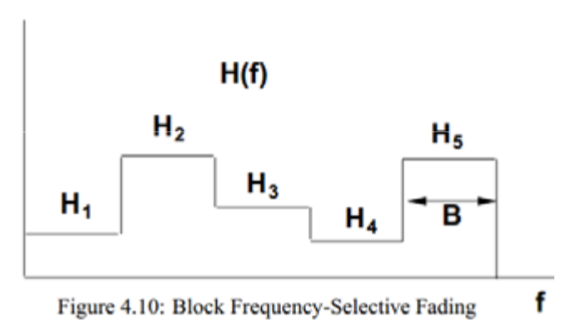容量：
$C=\sum_{\max P_{j}: \sum_{j} P_{j} \leq P} B \log _{2}\left(1+\frac{\left|H_{j}\right|^{2} P_{j}}{N_{0} B}\right)$
功控采用频率注水：
$\frac{P_{j}}{P}=\left\{\begin{array}{ll} \frac{1}{\gamma_{0}}-\frac{1}{\gamma_{j}} & \gamma_{j} \geq \gamma_{0} \\ 0 & \gamma_{j}<\gamma_{0} \end{array}\right.$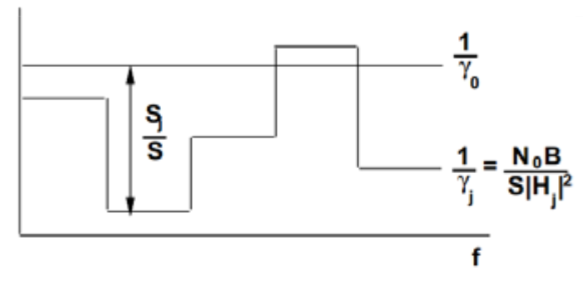时变
先在频率上用相关带宽切开，频率之间用注水，得到的每一块在时间上用注水（二维注水）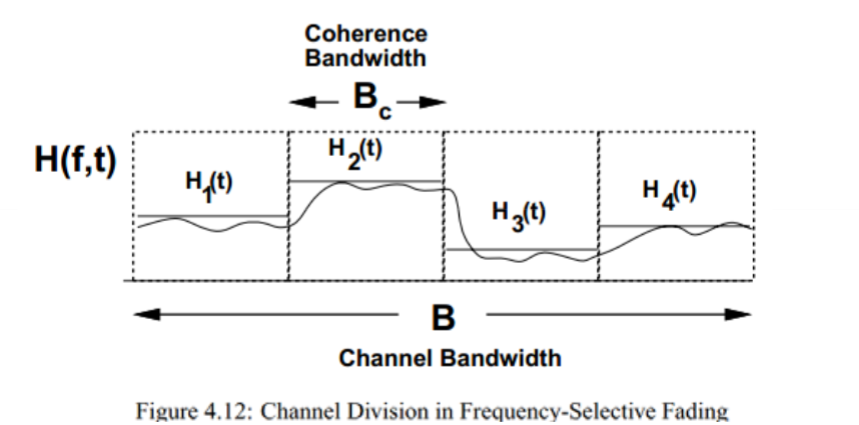展开全文• ## 信道容量的概念

千次阅读 2011-02-11 17:24:00
<br />http://jpkc.nwpu.edu.cn/dzjc/xiandaitongxin/jc/text/2.6.htm#lxtxxt    当一个信道受到加性高斯噪声的干扰时，如果... 1、信道容量的定义  在信息论中，称信道无差错传输信息的最大


http://jpkc.nwpu.edu.cn/dzjc/xiandaitongxin/jc/text/2.6.htm#lxtxxt

当一个信道受到加性高斯噪声的干扰时，如果信道传输信号的功率和信道的带宽受限，则这种信道传输数据的能力将会如何？这一问题，在信息论中有一个非常肯定的结论――高斯白噪声下关于信道容量的山农（Shannon）公式。本节介绍信道容量的概念及山农定理。
1、信道容量的定义
在信息论中，称信道无差错传输信息的最大信息速率为信道容量，记为。    从信息论的观点来看，各种信道可概括为两大类：离散信道和连续信道。所谓离散信道就是输入与输出信号都是取值离散的时间函数；而连续信道是指输入和输出信号都是取值连续的。可以看出，前者就是广义信道中的编码信道，后者则是调制信道。    仅从说明概念的角度考虑，我们只讨论连续信道的信道容量。
2. 山农公式
假设连续信道的加性高斯白噪声功率为（W），信道的带宽为（Hz），信号功率为（W），则该信道的信道容量为这就是信息论中具有重要意义的山农公式，它表明了当信号与作用在信道上的起伏噪声的平均功率给定时，具有一定频带宽度的信道上，理论上单位时间内可能传输的信息量的极限数值。    由于噪声功率与信道带宽有关，故若噪声单边功率谱密度为（W/Hz），则噪声功率。因此，山农公式的另一种形式为由上式可见，一个连续信道的信道容量受、、三个要素限制，只要这三个要素确定，则信道容量也就随之确定。
3. 关于山农公式的几点讨论
山农公式告诉我们如下重要结论：    （1）在给定、的情况下，信道的极限传输能力为，而且此时能够做到无差错传输（即差错率为零）。这就是说，如果信道的实际传输速率大于值，则无差错传输在理论上就已不可能。因此，实际传输速率一般不能大于信道容量，除非允许存在一定的差错率。    （2）提高信噪比（通过减小或增大），可提高信道容量。特别是，若，则，这意味着无干扰信道容量为无穷大；    （3）增加信道带宽，也可增加信道容量，但做不到无限制地增加。这是因为，如果、一定，有（4）维持同样大小的信道容量，可以通过调整信道的及来达到，即信道容量可以通过系统带宽与信噪比的互换而保持不变。例如，如果=7，=4000Hz，则可得=l2×b/s；但是，如果=l5，=3000Hz，则可得同样数值值。这就提示我们，为达到某个实际传输速率，在系统设计时可以利用山农公式中的互换原理，确定合适的系统带宽和信噪比。    通常，把实现了极限信息速率传送（即达到信道容量值）且能做到任意小差错率的通信系统，称为理想通信系统。山农只证明了理想通信系统的“存在性”，却没有指出具体的实现方法。但这并不影响山农定理在通信系统理论分析和工程实践中所起的重要指导作用。


展开全文• 考虑如下简单的加性高斯信道： 其中，X为离散输入符号集X=[a0,a1,⋯ ,aM−1]X ...根据信道容量的定义： C=max⁡{p(x=ai),i=1,2,...,M−1}{I(X;Y)}C = \mathop {\max }\limits_{\left\{ {p\left( {x = {a_i}} \right),
文章目录任意输入分布等概总结
任意输入分布
考虑如下简单的加性高斯信道：其中，X为离散输入符号集$X = \left[ {{a_0},{a_1}, \cdots ,{a_{M - 1}}} \right]$,由上图，$Y = X + n$。
根据信道容量的定义：
$C = \mathop {\max }\limits_{\left\{ {p\left( {x = {a_i}} \right),i = 1,2,...,M - 1} \right\}} \left\{ {I\left( {X;Y} \right)} \right\}$
可见，我们的目的是找到一个任意可能的输入信号的概率分布，使得最大化的互信息达到信道容量。如下图计算过程：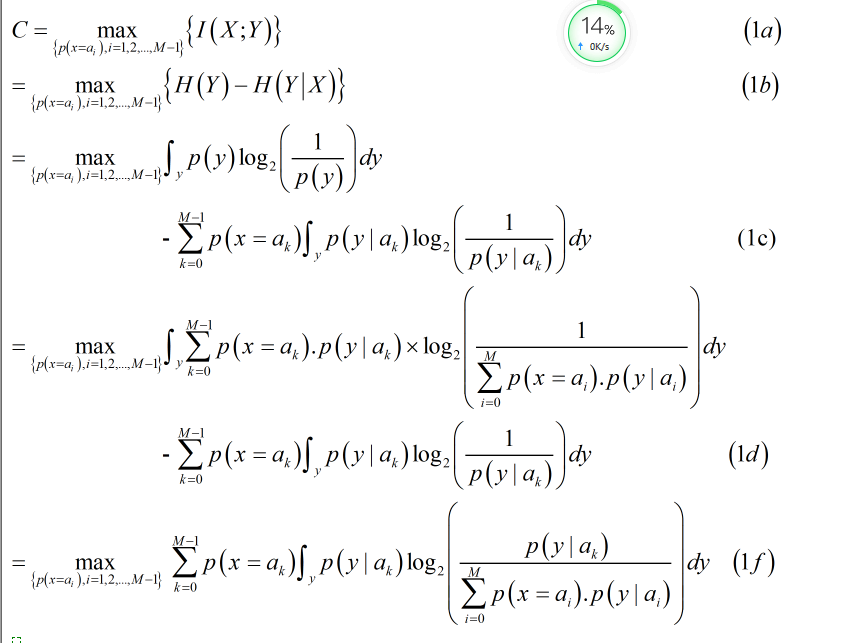其中，(1a)到(1c)是信息论课本中的相关定义，具体可百度；(1c)上半部分到(1d)是全概率公式的展开。
等概
假设输入符号是在M上是均匀分布的，
所以有：
$C = \frac{1}{M}\sum\limits_{k = 0}^{M - 1} {\int_y {p\left( {y|{a_k}} \right)} {{\log }_2}\left( {\frac{{p\left( {y|{a_k}} \right)}}{{\frac{1}{M}\sum\limits_{i = 0}^{M - 1} {p\left( {y|{a_i}} \right)} }}} \right)dy{\rm{ }}} （2）$
再用log(ab)=log(a)+log(b)与log(a/b) = -log(b/a)的性质，把上式分母中的1/M提出来，并将分数项倒过来，有：
$C = \frac{1}{M}{\log _2}M\sum\limits_{k = 0}^{M - 1} {\int_y {p\left( {y|{a_k}} \right)} } dy - \frac{1}{M}\sum\limits_{k = 0}^{M - 1} {\int_y {p\left( {y|{a_k}} \right)} } {\log _2}\left( {\frac{{\sum\limits_{i = 0}^{M - 1} {p\left( {y|{a_i}} \right)} }}{{p\left( {y|{a_k}} \right)}}} \right)dy{\rm{ }}（3）$
又因为,对该项$\int\limits_y {p\left( {y|{a_k}} \right)} dy$，其积分结果为1，这是因为归一性，即，y的所有概率和为1。所以有：
$C = {\log _2}M - \frac{1}{M}\sum\limits_{k = 0}^{M - 1} {\int_y {p\left( {y|{a_k}} \right)} } {\log _2}\left( {\frac{{\sum\limits_{i = 0}^{M - 1} {p\left( {y|{a_i}} \right)} }}{{p\left( {y|{a_k}} \right)}}} \right)dy{\rm{ }} （4）$
因为噪声是服从均值为0，方差为${\sigma ^2}$的高斯分布。对于p(y|ak)
有：
$p\left( {y|{a_k}} \right) = \exp \left( { - \frac{{{{\left| {y - {a_k}} \right|}^2}}}{{2{\sigma ^2}}}} \right) （5）$
将(5)带入(4)中，有：
$C = {\log _2}M - \frac{1}{M}\sum\limits_{k = 0}^{M - 1} {\int_y {p\left( {y|{a_k}} \right)} } {\log _2}\sum\limits_{i = 1}^{M - 1} {\exp \left( { - \frac{{{{\left| {y - {a_i}} \right|}^2} - {{\left| {y - {a_k}} \right|}^2}}}{{2{\sigma ^2}}}} \right)dy} （6）$
接着，令n=y-x,则dy=dz，y与z同号，故可进行积分代换,因为：故可以得到以下计算过程：其中，（6）到（7）是把y=x+n待入公式中，（7）到（8）是把积分写成数学期望的形式。（8）式的表达形式常可以在一些论文中见到。
总结

附：以上计算结果为单用户单天线的高斯信道，信道矩阵归一化为1了。可以参考经典论文，讨论的是单用户mimo高斯信道：

Globally Optimal Linear Precoders for Finite Alphabet Signals Over
Complex Vector Gaussian Channels

2.可以想象，如果是干扰信道的话，公式6中的y-ak不会再单纯的等于噪声n了，会引入干扰项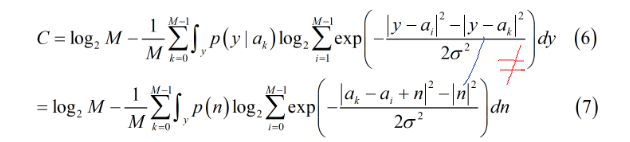具体是什么样的，后面我会再更新博客，也可以去搜一下关于广播和干扰信道的论文，其实就是广义二次矩阵相减的形式.
此外，在广播或者干扰信道的条件下，条件概率也不再单纯等于噪声，需要经过一点点小计算，最终的结果也会是用噪声来进行积分代换的。
敲公式不易，觉得有帮助的话麻烦点个赞把！


展开全文• 信道容量的定义为： C=I(X;Y)max⁡ C = I(X;Y)_{\max} C=I(X;Y)max​ 求解信道容量，即在以输入分布为自变量的情况下，求解平均互信息的极大值。需要注意的是：输入分布是信源概率空间中的概率，需要满足概率空间...
一种基于线性方程组的信道容量求解方法
1. 信道容量的一般性结论
信道容量的定义为：
$C = I(X;Y)_{\max}$
求解信道容量，即在以输入分布为自变量的情况下，求解平均互信息的极大值。需要注意的是：输入分布是信源概率空间中的概率，需要满足概率空间完备性。而直接通过驻点求解出的结论未必满足这一完备性。因此：信道容量的求解问题本质上是多元函数求解约束极值的问题。
1.1. 拉格朗日乘子法
多元函数的约束极值一般通过拉格朗日乘子法求解。拉格朗日乘子法的使用方法如下：

对于某多元函数$y=f(x_1,x_2,\cdots,x_n)$，需要求解其在$\Phi(x_1,x_2,\cdots,x_n)=0$条件下的极值；
构建函数：（其中参数$\lambda$称为拉格朗日乘子）
$g(x_1,x_2,\cdots,x_n,\lambda) = f(x_1,x_2,\cdots,x_n)-\lambda\Phi(x_1,x_2,\cdots,x_n)$
求解函数$g(x_1,x_2,\cdots,x_n,\lambda)$的极值，使该函数达到极值是函数$f(x_1,x_2,\cdots,x_n)$达到极值的必要条件。

在本问题的求解中，待求极值的多元函数是信道的平均互信息，约束条件是输入分布概率之和为一。于是构造函数的形式为：
$g(\vec{p_x},\lambda) = I(X;Y) - \lambda\left(\sum_ip(x_i) - 1\right)$
1.2. 求解驻点条件
互信息可以通过信源熵和信道疑义度，或者信宿熵与噪声熵表示。由于信道转移概率一般定义为以输入符号为条件关于输出符号的条件概率，因此使用信宿熵与噪声熵的表达式更方便：
$I(X;Y) = H(Y) - H(Y|X)$
信宿熵的求解基于信宿的概率分布特性。需要注意的是：信宿符号之所以表现出随机性，是因为信源符号分布和信道转移特性表现出了随机性，信宿的所有随机性来源于信源和信道，因此：
$p(y) = \sum_ip(x_i,y) = \sum_ip(y|x_i)p(x_i)$
由此可以得到信宿熵的表达式：
\begin{aligned} H(Y) &= -\sum_{j}p(y_i)\log_2p(y_i)\\ &= -\sum_j\left\{ \sum_ip(y_j|x_i)p(x_i) \times \log_2\left[\sum_ip(y_j|x_i)p(x_i)\right] \right\} \end{aligned}
噪声熵可以通过信道转移概率和信源分布表示：
\begin{aligned} H(Y|X) &= -\sum_{i,j}p(x_i,y_j)\log_2p(y_j|x_i)\\ &= \sum_ip(x_i)\left[ -\sum_jp(y_j|x_i)\log_2p(y_j|x_i) \right] \end{aligned}
要求解函数$g(\vec{p_x},\lambda)= I(X;Y) - \lambda\left(\sum_ip(x_i) - 1\right)$的极值，需要求解使得该函数达到驻点的自变量。这里将偏导求解按三项分别进行：

求解$- \lambda\left(\sum_ip(x_i) - 1\right)$关于$p(x_t)$的偏导：
该项是若干$p(x_i)$的累加，对$p(x_t)$求偏导时，只有包含$p(x_t)$的项才对导函数有作用，因此：
$\frac{\partial}{\partial p(x_t)}\left[- \lambda\left(\sum_ip(x_i) - 1\right)\right] = -\lambda$
求解$I(X;Y)$中噪声熵$H(Y|X)$关于$p(x_t)$的偏导：
该偏导的求解与上一项的求解过程类似，$H(Y|X)$也是一个关于$p(x_i)$各项的和式：
\begin{aligned} \frac{\partial}{\partial p(x_t)}H(Y|X) &= \frac{\partial}{\partial p(x_t)}\left\{ \sum_ip(x_i)\left[ -\sum_jp(y_j|x_i)\log_2p(y_j|x_i) \right] \right\}\\ &= -\sum_jp(y_j|x_t)\log_2p(y_j|x_t) \end{aligned}
求解$I(X;Y)$中信宿熵$H(Y)$关于$p(x_i)$的偏导：
\begin{aligned} \frac{\partial}{\partial p(x_t)}H(Y) &= \frac{\partial}{\partial p(x_t)} \left\{ -\sum_j\left\{ \sum_ip(y_j|x_i)p(x_i) \times \log_2\left[\sum_ip(y_j|x_i)p(x_i)\right] \right\} \right\}\\ &= -\sum_j\left[ p(y_j|x_t)\log_2p(y_j) + \frac{p(y_j|x_t)}{p(y_j)\ln2}\times p(y_j) \right]\\ &= -\sum_j\left[ p(y_j|x_t)\log_2p(y_j) + \frac{p(y_j|x_t)}{\ln2} \right] \end{aligned}

由此，得到对于$x_t\in X$，函数$g\left[\vec{p(x_t)},\lambda\right]$的导函数：
\begin{aligned} \frac{\partial g\left[\vec{p(x_t)},\lambda\right]}{\partial p(x_t)} &= -\sum_j\left[ p(y_j|x_t)\log_2p(y_j) + \frac{p(y_j|x_t)}{\ln2} \right] + \sum_jp(y_j|x_t)\log_2p(y_j|x_t) - \lambda\\ &= \sum_j\left\{p(y_j|x_t)\left[\log_2p(y_j|x_t) - \log_2p(y_j)\right]\right\} - \frac{1}{\ln 2}\sum_j p(y_j|x_t ) - \lambda\\ &= -\sum_j\left[p(y_j|x_t)\log_2\frac{p(y_j)}{p(y_j|x_t)}\right] - \left( \log_2e + \lambda \right)\\ \end{aligned}
在函数达到驻点时，导函数为零，于是有：
\left\{ \begin{aligned} \sum_ip(x_i) &= 0\\ -\sum_jp(y_j|x_t)\log_2\frac{p(y_j)}{p(y_j|x_t)} &= \left( \log_2e + \lambda \right) \end{aligned} \right.
1.3. 平均互信息达到信道容量时的性质
理论上，（1.2.）中得到的结论已经足以求解达到信道容量状态下的信源分布，但该表达式形式复杂，且并不是线性方程组，难以保证具有解析解。因此，在本文介绍的求解方法中，不使用该结论直接求解信源分布，而是通过该结论考察平均互信息达到信道容量时的性质，进而试图通过其他方法求解。
通过列写平均互信息的表达式：
\begin{aligned} I(X;Y) &= H(Y) - H(Y|X)\\ &= -\sum_j\left\{ \sum_i\Big[p(y_j|x_i)p(x_i)\Big] \times \log_2p(y_j) \right\} + \sum_ip(x_i)\left[ \sum_jp(y_j|x_i)\log_2p(y_j|x_i) \right]\\ &= -\sum_i\left\{ \sum_j\Big[p(y_j|x_i)p(x_i)\Big] \times \log_2p(y_j) \right\} + \sum_ip(x_i)\left[ \sum_jp(y_j|x_i)\log_2p(y_j|x_i) \right]\\ &=-\sum_ip(x_i)\left[\sum_jp(y_j|x_i) \times \log_2p(y_j) \right] + \sum_ip(x_i)\left[ \sum_jp(y_j|x_i)\log_2p(y_j|x_i) \right]\\ &= -\sum_{i}p(x_i)\left[ \sum_j p(y_j|x_i)\log_2\frac{p(y_j)}{p(y_j|x_i)} \right] \end{aligned}
当平均互信息达到信道容量时，有$-\sum_jp(y_j|x_t)\log_2\frac{p(y_j)}{p(y_j|x_t)} = \left( \log_2e + \lambda \right)$，于是：
$C = \log_2e+\lambda$
或者写成没有待定系数的形式：
$C = -\sum_jp(y_j|x_t)\log_2\frac{p(y_j)}{p(y_j|x_t)}$
2. 通过线性方程组求解信道容量
根据（1.3.）得出的结论：
$C = -\sum_jp(y_j|x_t)\log_2\frac{p(y_j)}{p(y_j|x_t)}$
这个表达式中，只有$C$、$p(x_t)$是未知数。需要注意的是，该表达式并不是一个方程，而是$\forall x_t \in X$，该方程都成立，即$s$个方程（$s$是信源符号的数量）。
根据对数函数的性质，可以将对数运算下的除法转换成对数运算外的减法，以便尽可能使用线性运算表达方程：
$C = \sum_jp(y_i|x_t)\log_2p(y_i|x_t) - \sum_jp(y_j|x_t)\log_2p(y_i)$
由于转移概率对于特定输入具有完备性，因此将信道容量也转换为乘以转移概率因子并求和的形式：
$\sum_jp(y_j|x_t)C = \sum_jp(y_i|x_t)\log_2p(y_i|x_t) - \sum_jp(y_j|x_t)\log_2p(y_i)$
至此，方程转变为：
$\sum_jp(y_j|x_t)\Big[ C +\log_2p(y_j) \Big] = \sum_jp(y_j|x_t)\log_2p(y_j|x_t)$
对于输入和输出状态相等的信道，$n = s = r$。定义未知数向量：
$\vec{\beta} = \begin{bmatrix} C+\log_2p(y_1)\\ C+\log_2p(y_2)\\ \cdots\\ C+\log_2p(y_n) \end{bmatrix}$
于是有：
$\mathbf{P}\vec{\beta} = \vec{H}$
其中$\mathbf{P}$是信道转移矩阵，$\vec{H}$是转移矩阵每行的转移概率组成的概率空间的熵，即：
$\vec{H} = \begin{bmatrix} \sum_jp(y_j|x_1)\log_2p(y_j|x_1)\\ \sum_jp(y_j|x_2)\log_2p(y_j|x_2)\\ \cdots\\ \sum_jp(y_j|x_n)\log_2p(y_j|x_n) \end{bmatrix}$
当$\mathbf{P}$是可逆矩阵时，可以通过矩阵运算求解出未知数向量：
$\vec{\beta} = \mathbf{P}^{-1}\vec{H}$
根据信宿熵的完备性，可以通过$\vec{\beta}$向量求解信道容量$C$：
$\sum_j2^{C+\log_2p(y_j)} = \sum_j2^cp(y_j) = 2^c$
于是解得信道容量为：
$C = \log_2\left(\sum_j2^{\beta_j}\right)$


展开全文数字通信 数学 矩阵
• 在不同《信息论》教材中，有关信道对称性描述并不统一，这为学习对称性信道的信道容量计算方法造成一定障碍。因此，本文在文章开篇部分将对本文中所描述三种具有对称性信道进行严格定义，以减小文章出现...
• 当一个信道受到加性高斯噪声的干扰时，如果信道传输信号的功率和信道的带宽受限，则这种信道传输... 1、信道容量的定义 在信息论中，称信道无差错传输信息的最大信息速率为信道容量，记为。 从信息论的观点来看，
• ## MIMO的信道容量以及实现

千次阅读 多人点赞 2019-09-28 14:39:25
所谓信道容量，是指信道能无差错传输信息最大信息速率，从信息论角度，信道容量定义为： C=max⁡f(x) I(x,y)C=\underset{f(x)}{\mathop{\max }}\,I(x,y)C=f(x)max​I(x,y)      &...
• %一维二元高斯信道的信道容量xn=8000;%定义点数m1=0;m2=1;%信号参数sigma1=0.38;sigma2=0.5;%高斯噪声参数xmin=min([m1-5*sigma1,m2-5*sigma2]);xmax=max([m1+5*sigma1,m2+5*sigma2]);%定义域边界x=linspace(xmin,...
• 我们平常所说“带宽”不是带宽，而是信道容量信道容量与带宽设计到两个公式 1.尼奎斯特公式 2.香浓公式 信道要搞清楚以上两个定理，我们要先弄懂一些术语定义：波特率（baud rate）、比特率（bit rate）、...
• 信道噪声 1.加性噪声 加性噪声(Additive noise)是信道中存在不需要电信号，它独立于信号始终存在。 影响：加性噪声会使模拟信号失真，数字信号发生错码，限制信息传输速率。 2.加性噪声分类 按噪声...
• 一些公式没法粘贴，以后再补上  一、用了matlab实现DMC容量迭代的算法如下： 设信道输入和输出符号集大小分别为r,s. ...二、了解了信道容量的定义和DMC信道容量迭代计算方法，我用了matlab来进行编程进行
• 数据传输速率的定义 ：带宽与数据传输速率 ： 低通信道： 无线电入门的一些基础问题
• 今天我们给出了有关 MIMO 信道容量理论推导更多细节。利用 h (τk, t) 定义在时间 t 和时延 τk 处信道脉冲响应，可以采用一个线性时变系统作为无线信道模型，其中 k = 0，...，L-1，L 为多径编号。用 hi,j ...随机矩阵理论
• 根据极化码原始论文中提出的信道容量为 它是一种信道速率丈量,标志着信道速率上限，，即信道输入与输出互信息量最大值； 所谓互信息定义是： 设两个随机变量联合分布为，边缘分布分别为，互...
• #include #include #define MAX 9 int main() { /*定义数组及中间变量*/ double fun(int n,double g[MAX][MAX]); double b[MAX]={0},d[MAX]={0},t[MAX][MAX]={{0}},g[MAX]={0},h[MAX]={{0}};
• 今天我们给出了有关 MIMO 信道容量理论推导更多细节。利用 h (τk, t) 定义在时间 t 和时延 τk 处信道脉冲响应，可以采用一个线性时变系统作为无线信道模型，其中 k = 0，...，L-1，L 为多径编号。用 hi,j ...
• 针对矿井巷道,给出了一种M IMO相关性信道建模方法。...通过MATLAB仿真,将相关信道和瑞利信道容量累计概率分布对比,证明了相关性存在降低了信道容量,增加天线数目和间距可以降低相关系数,增加信道容量
• matlab仿真mimo信道容量 SNR=15; %定义信噪比为0dB disp('当nT=4时'); %显示接收天线数等于1 A=10^(SNR/10); %信噪比单位转换关系式 Imin=eye(4); %"1"为发射天线和接收天线两者数目较少根...
• 我们都是到对于任何一个信号经过傅里叶变换（Fourier Transition），可以在一个频率谱第一个零点定义为信号带宽，常常被我们认为信号基带带宽（Base bandwidth）。基带信号在发射过程中经过信号调制后根据信道的...
• 波特率极限波特率或码元率或调制速率，其定义是：通信系统每单位时间内传输码元脉冲个数；或者信号调制过程中，每单位时间内调制信号波形变换次数（基带信号都是低频信号，必须调制到高频微波、毫米波信号，才能...
• 信道及其容量的问题 信道的定义是广义的，可以指一段线路，也可以包含了设备的复杂系统。若信道的输入为X=(X1,X2，......，Xi，......)，输出为Y=(Y1,Y2，......，Yi，......)，通常定义条件概率p(Y|X)来描述信道...c
• 信道容量定义为信道输入与输出平均互信息最大值。信道容量仅于信道特性，信道转移概率有关，与信道输入无关。但是信道输入概率必须与信道容量进行匹配才能达到容量。 2. 单符号离散信道 一个平稳离散无记忆信道...
• MIMO MB-IR-UWB系统信道容量分析，金子靖，窦峥，文章从MIMO MB-IR-UWB系统工作模式入手，根据系统子波段选取规则不同定义了四种不同系统工作模式，即IR-UWB/1b×1模式、1b×Nt模式、Lb×1��
• 考虑MIMO信道的一般表达式： $$Y= HX +N \qquad (1)$$ 其中 $$X-H_t \times 1$$ $$Y-H_r \times 1$$ $$X,N- i.i.d Gaussian Distribution$$ 同时定义 $$R_{xx}= E[XX^H]$$  R_{nn}= E[NN^H] = \sigma...
• 信道容量 一. 信息量 1. 定义 信息量是对事件不确定性度量，单位bit。 定义：在随机变量X中，事件x(自)信息量I(X=x)I(X=x)I(X=x)简写为I(x)=−log2p(x)I(x)=−log_2 p(x)I(x)=−log2​p(x)，单位bitbitbit。 ...数字通信
• 我们都是到对于任何一个信号经过傅里叶变换(Fourier Transition)，可以在一个频率谱第一个零点定义为信号带宽，常常被我们认为信号基带带宽(Base bandwidth)。基带信号在发射过程中经过信号调制后根据信道的宽度...
• 写在前面：本文源自笔者在大三时对北师大-人工智能...1）信道的定义：连接发送端和接收端通信设备之间的传输媒介，信号传输的通道 2）信道的分类： ① 按传输媒介分类： - 有线信道：明线、电缆（对称电缆、同轴电...数字通信...